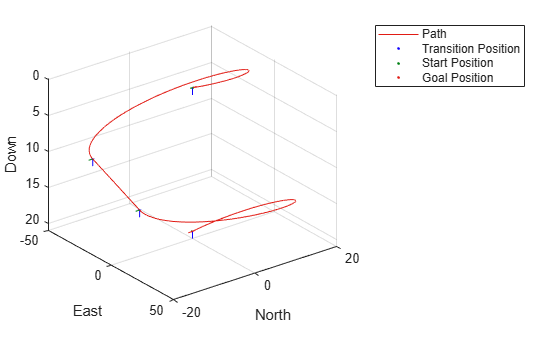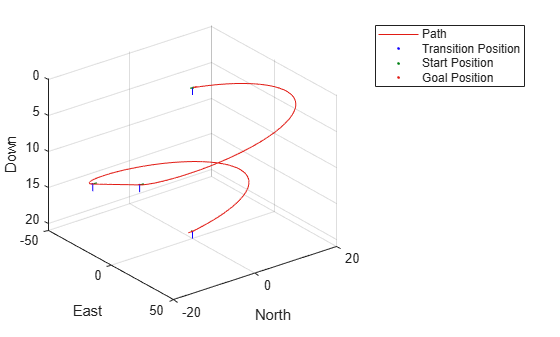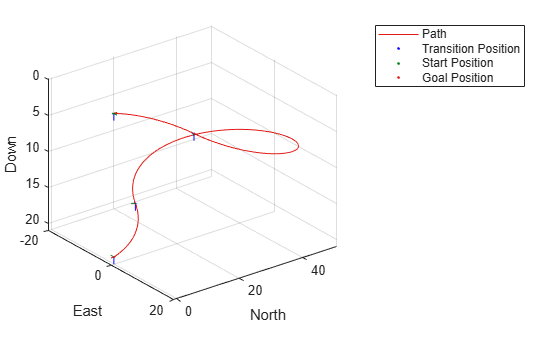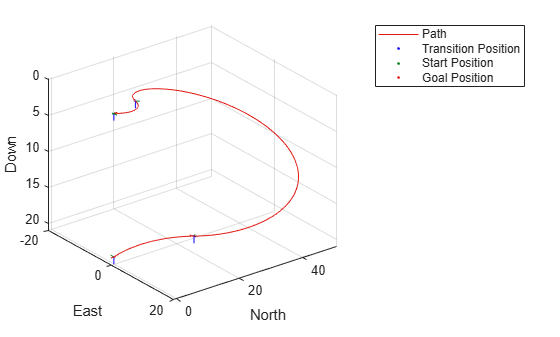# connect

Connect poses with UAV Dubins connection path

Since R2019b

## Syntax

``[pathSegObj,pathCost] = connect(connectionObj,start,goal)``
``[pathSegObj,pathCost] = connect(connectionObj,start,goal,'PathSegments','all')``

## Description

````[pathSegObj,pathCost] = connect(connectionObj,start,goal)` connects the start and goal poses using the specified `uavDubinsConnection` object. The path segment object with the lowest cost is returned.```

example

````[pathSegObj,pathCost] = connect(connectionObj,start,goal,'PathSegments','all')` returns all possible path segments as a cell array with their associated costs.```

## Examples

collapse all

This example shows how to calculate all valid UAV Dubins path segments and connect poses using the `uavDubinsConnection` object.

Calculate All Possible Path Segments

Create a `uavDubinsConnection` object.

`connectionObj = uavDubinsConnection;`

Define start and goal poses as [`x`, `y`, `z`, `headingAngle`] vectors.

```startPose = [0 0 0 0]; % [meters, meters, meters, radians] goalPose = [0 0 20 pi];```

Calculate all possible path segments and connect the poses.

`[pathSegObj,pathCosts] = connect(connectionObj,startPose,goalPose,'PathSegments','all');`

Path Validation and Visualization

Check the validity of all the possible path segments and display the valid paths along with their motion type and path cost.

```for i = 1:length(pathSegObj) if ~isnan(pathSegObj{i}.Length) figure show(pathSegObj{i}) fprintf('Motion Type: %s\nPath Cost: %f\n',strjoin(pathSegObj{i}.MotionTypes),pathCosts(i)); end end``````Motion Type: L S L N Path Cost: 214.332271 ``````Motion Type: R S R N Path Cost: 214.332271 ``````Motion Type: R L R N Path Cost: 138.373157 ``````Motion Type: L R L N Path Cost: 138.373157 ```

## Input Arguments

collapse all

Path connection type, specified as a `uavDubinsConnection` object. This object defines the parameters of the connection.

Initial pose of the UAV at the start of the path segment, specified as a four-element numeric vector or matrix [x, y, z, headingAngle].

x, y, and z specify the position in meters. headingAngle specifies the heading angle in radians. The heading angle is measured clockwise from north to east. Each row of the matrix corresponds to a different start pose.

The pose follows the north-east-down coordinate system.

The `start` and `goal` pose inputs can be any of these combinations:

• Single start pose with single goal pose.

• Multiple start poses with single goal pose.

• Single start pose with multiple goal poses.

• Multiple start poses with multiple goal poses.

Goal pose of the UAV at the end of the path segment, specified as a four-element numeric vector or matrix [x, y, z, headingAngle].

x, y, and z specify the position in meters. headingAngle specifies the heading angle in radians. The heading angle is measured clockwise from north to east. Each row of the matrix corresponds to a different goal pose.

The pose follows the north-east-down coordinate system.

The `start` and `goal` pose inputs can be any of these combinations:

• Single start pose with single goal pose.

• Multiple start poses with single goal pose.

• Single start pose with multiple goal poses.

• Multiple start poses with multiple goal poses.

## Output Arguments

collapse all

Path segments, returned as a cell array of `uavDubinsPathSegment` objects. The type of object depends on the input `connectionObj`. The size of the cell array depends on whether you use single or multiple `start` and `goal` poses.

By default, the function returns the path with the lowest cost for each `start` and `goal` pose.

When calling the `connect` function using the `'PathSegments','all'` name-value pair, the cell array contains all valid path segments between the specified `start` and `goal` poses.

Cost of path segments, returned either as a positive numeric scalar, vector, or matrix. Each element of the cost vector corresponds to a path segment in `pathSegObj`.

By default, the function returns the path with the lowest cost for each `start` and `goal` pose.

## Version History

Introduced in R2019b